# High School Chemistry : Identifying Mixtures and Solutions

## Example Questions

### Example Question #1 : Identifying Mixtures And Solutions

What is the molarity of a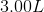solution that contains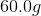of potassium nitrate?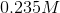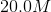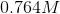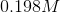Explanation:

In order to solve for molarity, we need to know the necessary equation to use. Molarity is given by the moles of solute divided by the liters of solution.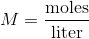Since we are given the mass of potassium nitrate, we must first convert it into moles before we can solve for the molarity of the solution. Potassium nitrate has the formula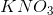. Use this to calculate the molar mass.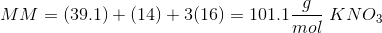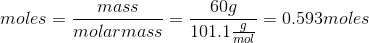Now that we have the number of moles, we can solve for the molarity of the solution using the given volume: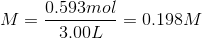### Example Question #1 : Solutions And Mixtures

Which of the following is an example of a mixture?

A sugar cube dissolved in a cup of coffee

A glass of warm salt water

A nutrient mix for plants contains dissolved nitrogen, phosphorus, and potassium

Yeast in the bottom of a bottle of wine

Yeast in the bottom of a bottle of wine

Explanation:

A mixture can be filtered or otherwise separated into its individual components. In this case, we could filter out the solid yeast from the bottom of the bottle of liquid wine. However, we could not filter out the salt, sugar, or nutrients since they are chemically mixed into the water, are in the same phase. The the salt water, coffee, and nutrient water are incorrect since these are solutions.

### Example Question #1 : Identifying Mixtures And Solutions

Which of the following is an example of a solution?

A farmhand combining solid fertilizers in a bin

Rice cooking in a pot of water

A chef making a salad out of spinach, walnuts, gorgonzola cheese, and apples

Explanation:

To identify a solution, look for a homogenous mixture composed of only one phase. Thre rice, salad, and fertilizer are descriptions of mixtures: combinations of materials which can be physically separated. The only option describing a solution is the lemonade solution since the concentrate is dissolved in water, which results in a homogenous mixture. Solutions are both mechanically and chemically combined.

### Example Question #2 : Identifying Mixtures And Solutions

Which is considered the solute when hydrochloric acid is used to disolve sodium bisulfite?

Hydrochloric acid

Sodium bisulfite

None of these is correct

Two or more of these are correct

Sodium bisulfite

Explanation:

The solvent can be thought of as the substance present in greater amount, or the substance in which the solute is dissolved. Solvents are often liquid. The solute is the substance present in lesser amounts, and is dissolved by the solvent. In this case, the hydrochloric acid is the solvent, because it dissolves the sodium bisulfite. Because it is getting dissolved, the sodium bisulfite is the solute.

### Example Question #11 : Solutions And Mixtures

Which phase label subscript is associated with a soluble compound?

(l)

(aq)

(r)

(s)

(g)